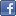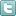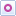EE Times-India > T&M

T&M# An overview of S-parameters

Posted: 12 Dec 2014Print Version

Keywords:electromagnetic radiation  scattering matrix  S-matrix  network  time-domain reflectometer measurements

Say, for instance, you have an optical lens of some sort onto which you shine a light with a known photonic output. While most of the incident light passes through the lens, some fraction of the light is reflected and some is absorbed (the behaviour is also dependent on the wavelength of the incident light). You'd like to characterise that lens: Exactly how much light was reflected? How much passed through? What is it about the lens that prevented all of the light from passing through?

Now, let's apply that same sort of thinking to a two-port network. When we apply a signal to the network's input, the same thing will happen as it did with the lens: Some fraction of the signal will propagate through the network but some is reflected, or scattered, back through the port it entered through. Some of the signal will be dissipated as heat, or even as electromagnetic radiation. As with the lens, we want to know what's happening with our two-port network. What is causing the scattering of the signal? Bear in mind that the "signal" is an electromagnetic wave propagating through the medium of the network, just as the light passing through the lens is also an electromagnetic wave.

The answer lies in the application of a mathematical construct called a scattering matrix (or S-matrix), which quantifies how energy propagates through a multi-port network. The S-matrix is what allows us to accurately describe the properties of incredibly complicated networks as simple "black boxes". The S-matrix for an N-port network contains N2 coefficients (S-parameters), each one representing a possible input-output path. By "multi-port network," we could be referring to, for example, a cable or a microstrip line. How would that interface affect a 5-Gbps USB 3.0 signal? This is the sort of practical application in which S-parameters shine.

S-parameters are a "frequency-domain" description of the electrical behaviour of a network. They are complex numbers, and are expressed in terms of both magnitude and phase. That's because both the magnitude and phase of the input signal are changed by the network due to losses, reflections, and propagation time. Quite often we refer to the magnitude only, because how much gain or loss occurs is typically of the most interest. However, the phase information is extremely important and should not be ignored. S-parameters are defined for a given set of frequencies and port impedance (typically 50Ω), and vary as a function of frequency for any real-world network.

There are also mixed-mode S-parameters. Say you have a differential lane in your circuit and you want to quantify the losses in a differential signal. S-parameters for the differential lane can be transformed into differential S-parameters, with which you consider differential signal characteristics alongside of common signal characteristics.

In their most basic sense, S-parameters refer to the ratio of "voltage out versus voltage in." S-parameters come in a matrix, with the number of rows and columns equal to the number of ports. For the S-parameter subscripts "ij", j is the port that is excited (the input port), and "i" is the output port. Thus, S11 refers to the ratio of signal that reflects from port one for a signal incident on port one, as a function of frequency. S-parameters along the diagonal of the S-matrix are referred to as reflection coefficients because they only refer to what happens at a single port, while off-diagonal S-parameters are referred to as transmission coefficients, because they refer to what happens from one port to another. Figure 1 shows the S-matrices for one, two, and three-port networks.Figure 1: The S-parameters for one, two, and three-port networks.

Comment on "An overview of S-parameters"

Top Ranked Articles

WebinarsVisit Asia Webinars to learn about the latest in technology and get practical design tips.

Search EE Times India
Services

Go to topConnect on FacebookFollow us on TwitterFollow us on Orkut
﻿